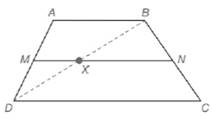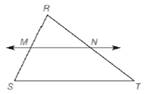Chapter 5.6, Problem 34E### Elementary Geometry for College St...

6th Edition
Daniel C. Alexander + 1 other
ISBN: 9781285195698

#### Solutions

Chapter
Section### Elementary Geometry for College St...

6th Edition
Daniel C. Alexander + 1 other
ISBN: 9781285195698
Textbook Problem
33 views

# Use Exercise 33 and the following drawing to complete the proof of this theorem: “The length of the median of a trapezoid is one-half the sum of the lengths of the two bases”. Given: Trapezoid A B C D with median M N ¯Prove: M N = 1 2 ( A B + C D ) 33. Use Theorem 5.6.1 and the drawing to complete the proof of this theorem: “If a line is parallel to one side of a triangle and passes through the midpoint of a second side, then it will pass through the midpoint of the third side.” Given: Δ R S T with M the midpoint of R S ; ¯ M N ↔ ∥ S T ¯Prove: N is the midpoint of R T ¯

To determine

To prove:

MN=12(AB+CD).

Explanation

Given:

We need to prove, “The length of the median of a trapezoid is one-half the sum of the lengths of the two bases”.

In a trapezoid ABCD, MN¯ is the median.

Property used:

Means-Extremes Property:

In a proportion, the product of the means equals the product of the extremes; that is, if ab=cd (where b0 and d0), then ad=bc.

If a line is parallel to one side of a triangle and intersects other two sides, then it divides these sides proportionally.

Proof:

Consider a trapezoid ABCD.

Given that MN¯ is the median.

We know that the median of the trapezoid is parallel to each base.

MN¯AB¯CD¯

We know that, if a line is parallel to one side of a triangle and passes through the midpoint of a second side, then it will pass through the midpoint of the third side

### Still sussing out bartleby?

Check out a sample textbook solution.

See a sample solution

#### The Solution to Your Study Problems

Bartleby provides explanations to thousands of textbook problems written by our experts, many with advanced degrees!

Get Started

#### Evaluate the limit, if it exists. limx4x2+95x+4

Single Variable Calculus: Early Transcendentals

#### True or False: is a convergent series.

Study Guide for Stewart's Multivariable Calculus, 8th

#### Graph each function. y=x+2

College Algebra (MindTap Course List)